scroll

# Parallel Computing and Multiprocessing in Python

We all know that completing a task together is much faster than doing it alone. A similar principle is true in the methodology of parallel computing. These calculations can be performed either by different computers together, different processors in one computer or by several cores in one processor. A separate case of parallel tasks is the use of the GPU. Let's look at the task of organizing parallel computing in Python. We will use a very simple computational task and a very simple method of parallel computation on processors to get the sample clear and intuitive code. Of course, there are other methods for organizing Python parallel processing and other programming languages and computer systems.

## What is Parallel Computing

Parallel computing is a data processing method in which one task is divided into parts and then each part is calculated on its device simultaneously. Then the results from all devices are connected together. Thus, you can quickly calculate a fairly large number of complex tasks. Of course, there is a limitation in the form of Amdahl's law

Parallel computing has evolved since the 1950s. It remains relevant even now when the processor in a mobile phone can have 16 or more cores and processors in budget home computer GPUs now number in the thousands.

There are two main paradigms for parallel computing: shared memory and message passing.

Parallel computing using shared memory allows multiple computing devices to access the same shared memory location. All devices can read from such a cell at the same time, but only one device can write at a time. Thus, from a programming point of view, it is a simple and convenient model.

Parallel computing via message passing allows multiple computing devices to transmit data to each other. Thus, an exchange is organized between the parts of the computing complex that work simultaneously. Such a model is quite common and is widely used in programming.

Both of these approaches have their advantages and disadvantages. The main problem in the implementation of parallel computing is the synchronization of parts of the algorithms, tracking the steps of computing and recording or transmitting data from various computing devices.

Multiprocessing is the process of using two or more central processing units (CPUs) in one physical computer. There are many options for multiprocessing; for example, several cores on one chip, several crystals in one package, several packages in one system unit, etc. A multiprocessor system is a computer device that has two or more processor units (several processors), each of which shares the main memory and peripheral devices for simultaneous processing of programs.

In computer architecture, multithreading is the ability of a central processing unit (CPU) (or a single core in a multi-core processor) to simultaneously provide multiple threads of execution. Multiprocessing vs parallel processing are similar approaches to make computation more effective. But multithreading is different from multiprocessing since, a multithreaded application, threads share the resources of one or more cores. These can be computed blocks, CPU caches, and a translation buffer (TLB) available to several threads simultaneously.

Please note that some Python interpreters can perform multithreading sequentially but not in parallel.  They are specific  Python threads that differ from other programming languages. You can find more information about the global interpreter lock (GIL) here

## How to perform Python parallel computing

Let's use Python as a programming language. This is an excellent language for writing various computational algorithms, especially since there are a lot of corresponding libraries for this.

Let's solve such a simple problem as finding the sum of all integers from 1 to 20 inclusive. A simple task, even for a single-processor system, but very visual. This task has no practical sense since parallel computing will be effective for a large data set. But in order to understand the concept of parallel computing, this example is great.

There are tons of approaches to organizing parallel computing in Python. One of them, the simplest, is to start several processes. Each process will count its part of the task. Then these two results will merge together into one result. How can such calculations be organized in several lines of code? Let's get a look.

Start a new notebook at Google Colab, a wonderful environment to try your hand at a parallel Python task. Let's print the version of Python that runs on Colab. To do this, work out this simple code: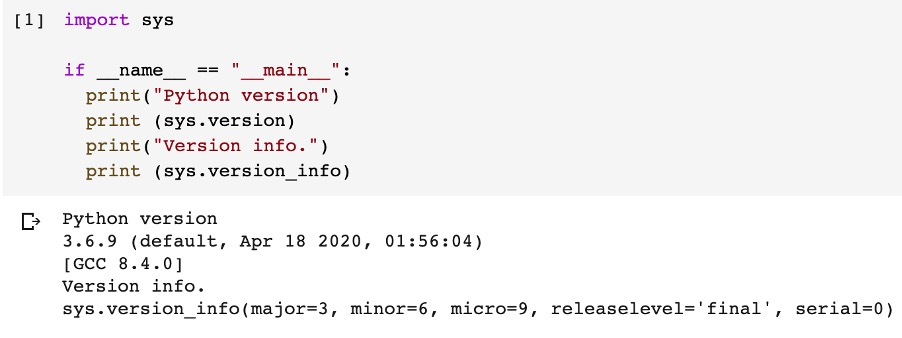Works great. And we have at our disposal Python version 3.6.9.

Then let’s take a look at Google Colab multiprocessing capabilities.

We will solve the problem of finding the sum of all integers from 1 to 20 inclusive. Let's write the framework of the function that will perform the calculations: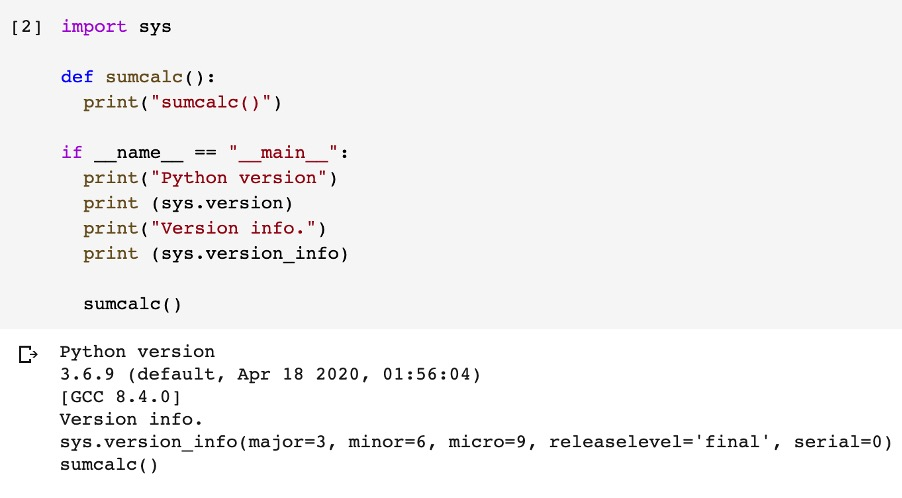And as you can see from the results of the work, this function is launched.

Let’s add multiprocessing to this code by including import multiprocessing. Then let’s find out how many CPUs we have in the system available my multiprocessing.cpu_count().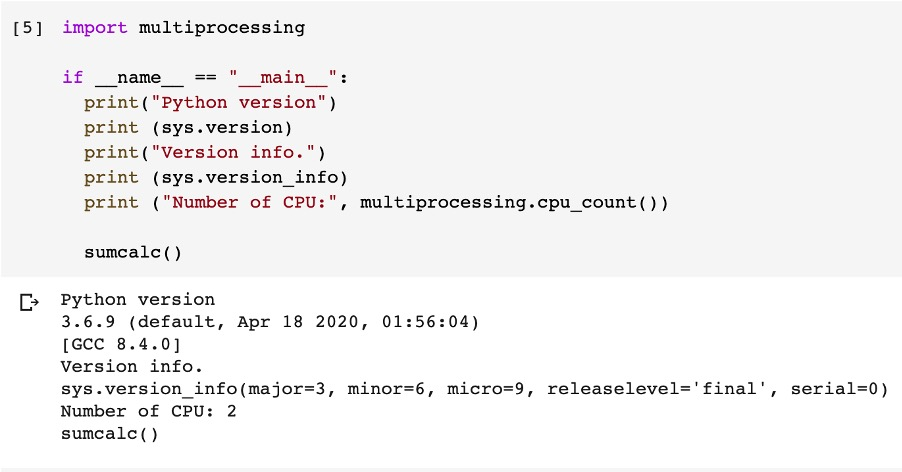Great, we have 2 CPU’s available in our Colab multiprocessing environment. This will allow us to run our code in parallel.

Let’s create function sumcalc(myrange) to calculate the sum of values in the given range.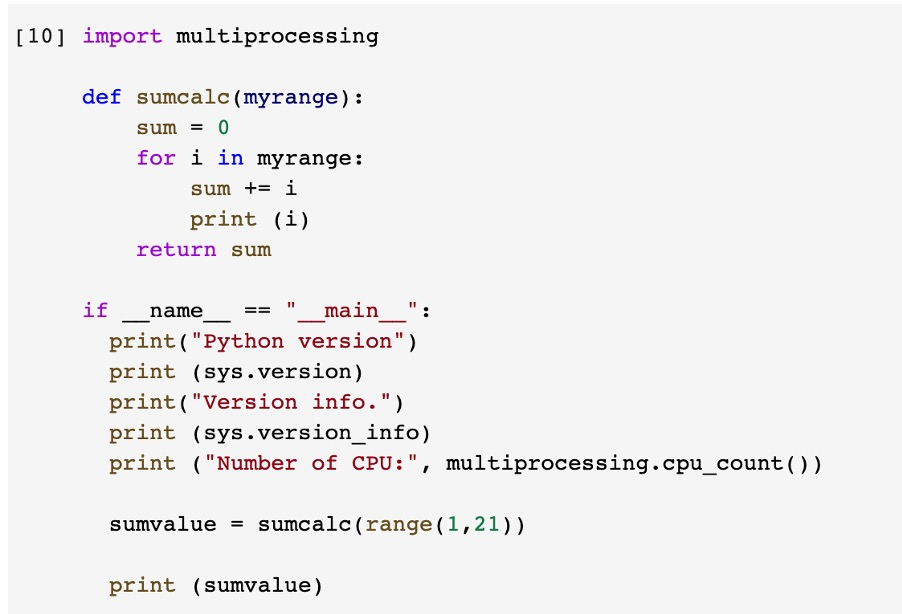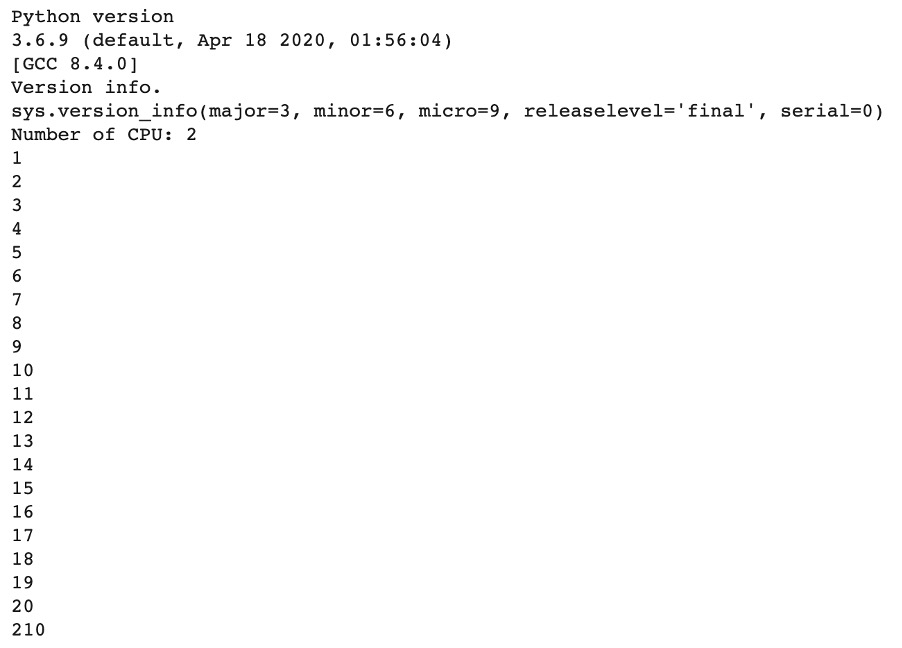Very good, this function calculates the sum of values from 1 to 20. The result of sum is 210.

Further, this function can be run twice at the same time, that is, in parallel. We will do this using multiprocessing. We will start each process using Process(target=sumcalc, args=(sumval, range(from,to))), where sumcalc is our function for calculating the sum. Next, use start() to start these processes in parallel. And then using the join() function, we will wait for each process to complete. This will enable us to wait until the process terminates.

But how to transfer some data to these functions?  Remember that the functions will be calculated simultaneously. To do this, we will use the args=() parameter and pass some value there. In the function itself, we will take this parameter at the input. Let's pass two parameters to the function. In our case, these will be the sum value and the range of the set of integers that we will add. That is, we transfer 1 and 11 to the first function, and 11 and 21 to the second. We take into account that 11 and 21 are not included in the amount.  Now we have two functions that in parallel give us numbers from 1 to 10 inclusive and from 11 to 20 inclusive, as we wanted.Let's now calculate the sum of all these numbers. There are many methods for doing this, but the simplest and most obvious way is to use shared memory, that is, one shared variable that will be available in two processes at once. There, each stream will add numbers from its range, digit by digit. We will write in the sumval = Value('d', 0.0) method from multiprocessing.Value so that each of the processes sees the total value of the sum. Value accepts type 'd' (double) and initial value 0.0: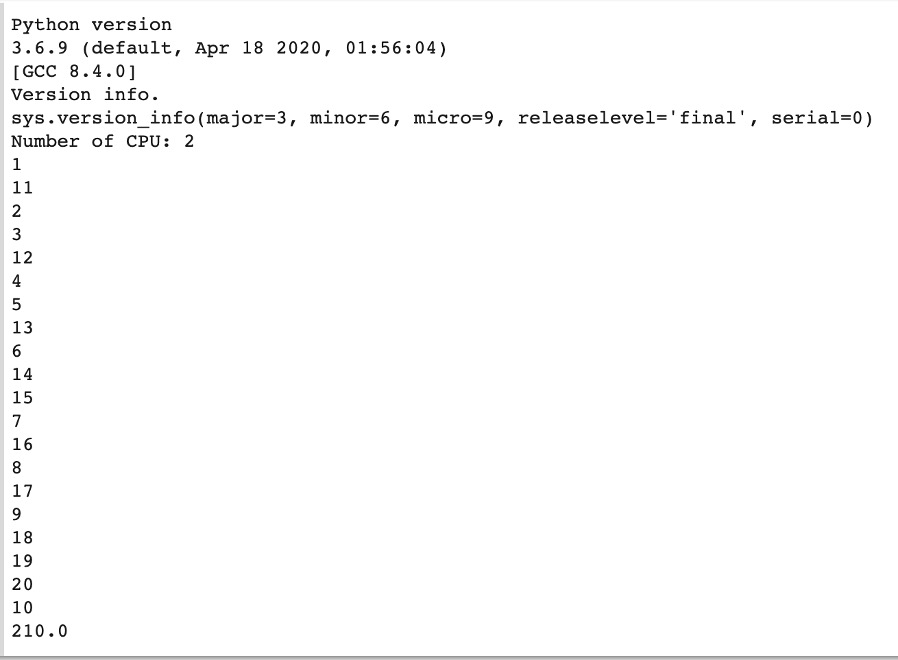Very good, Colab parallel processing works, and we got the result 210. And as you can see, values are printed in the way of parallel execution. To measure performance you can change the range of sum calculation by about 1-2 million and parallel performance will show the practical speedup.

## Unit testing of checking the result

Now we need to check whether the amount was calculated correctly. To do this, you can write a unit test. This is also quite simple to do. Include the line in the import unittest code. Then add the class TestSumMethods (unittest.TestCase). In this class, you can implement the test function test_sum, which calculates the sum of numbers from 1 to 20 inclusive. Next, using the assertEqual function, we compare the result we obtained from the parallel algorithm with our implementation of the unit test.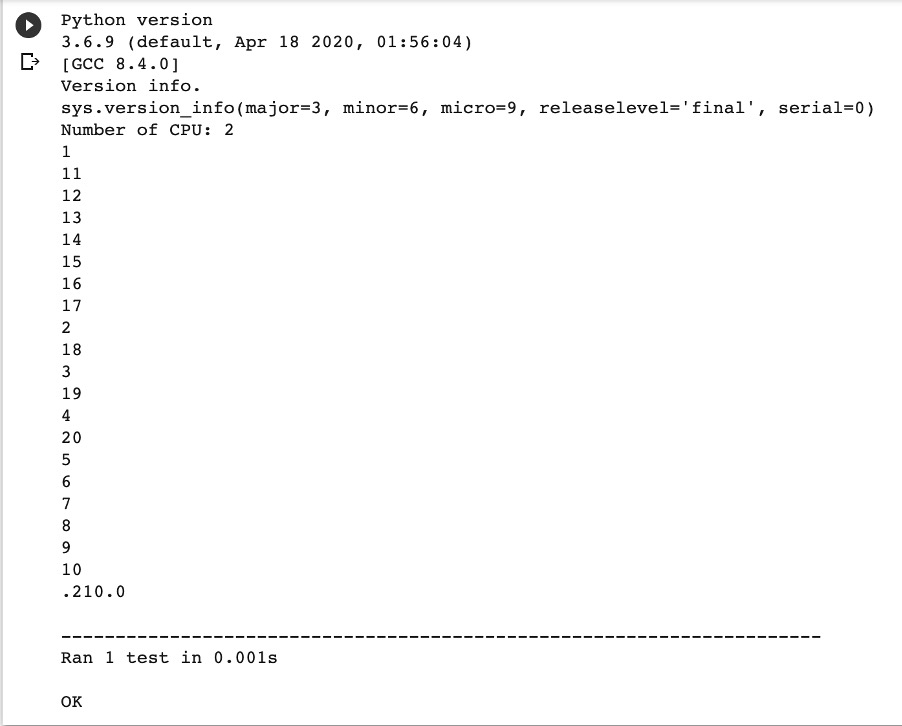After launching we see the results of the calculation in the test function and in the flows are the same. The code turned out to be not very complicated, but these are parallel computations, which many developers are afraid of. Of course, in large tasks, certain difficulties may arise with the synchronization of processes, catching certain processes from thousands of running ones, the redistribution of shared memory, and a number of other problems, but all this can be solved, as practice shows.

## Conclusion

In conclusion, I want to say that this example is very simple and specially selected to show that tasks even on Python can be considered parallel methods. You can familiarize yourself with parallel computing in Python at this link.

For a significant increase in the speed of code in Python, you can use Just In Time Compilation. Among the most famous systems for JIT compilation are Numba and Pythran. By the way, they also have special means of organizing multiprocessing computing, including the GPU.

Our specialists from Svitla Systems from all development departments always try to solve the problem in an optimal way. Developers, architects, and testers always plan to use the best and newest solutions exactly where they are needed, including parallel calculations. We develop solutions that will work reliably, sustainably, and optimally with minimal time and budget for development. This is precisely our company’s distinguishing feature, regardless of the applied programming languages, frameworks, or cloud systems.by Svitla Team